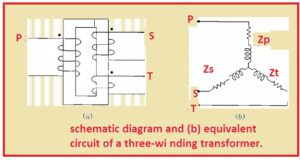Hello, readers welcome to new post. In this post, we will have a detailed look at Three Phase Transformer Per unit Impedance. Three phases transformer is the main element of the system and operates at mutual induction phenomenon. according to that flux change in first windings induced volts in second windings according to that linking flux to the secondary windings.

In this post, we discuss the three-phase transformer per unit impedance. SO let get started with

### Three Phase Transformer Per unit Impedance

• In the normal configuration of 2 windings transformer primary and secondary windings has a similar value of KVA but in the three-phase, these values are not same.• The impedance value of every winding of the transformer is given in per unit system or in percentage according to ratings.
• But there should be same value for base KVA in above two methods of impedance define.
• The design of the signal phase having three windings is shown. the windings are primary secondary and tertiary.
• A short circuit test is done to finds the impedance.
• ZPs is leakage impedance measured tough primary side having secondary s short circuit and tertiary as open.
• Zpt is primary impedance measured doing the short circuit at tertiary keeping secondary s open circuit
• Zst at secondary measured at tertiary short-circuited and primary as open circuit

Now we have

Zpt= Zp+Zt

Zps=Zp+Zs

Zst=Zs+Zt

• In the above equations Zp, Zs, and Zt are impedance values at primary secondary and tertiary windings.
• By simplifying the above equation we have

Zp=1/2(Zps+Zpt-Zst)

Zs=1/2(Zps+Zst-Zpt)

Zt=1/2(Zpt+Zst-Zps)

• The impedance of 3 windings 3 coils are linked to a resultant circuit of single-phase 3 windings transformer connected to magnetizing current.
• The points P s and t are linked to the three windings of the transformer.
• In case of 2 windings transformer, there is a need for a similar KVA base for 3 circuitry and needed voltage bases for 3 circuits having a similar ratio like the rated line to line voltage of the device.

That is al about the Three Windings Transformer Per unit Impedance if you have any further query ask in the comments. Thanks for reading have a nice day